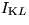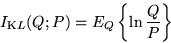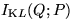Next: Model selection Up: Ensemble Learning for Independent Previous: Introduction

# Ensemble learning

Assume we would like to make a prediction, decision, etc., based on measurements and some kind of models. From the axioms of Bayesian probability theory it follows that all the models should be used in the process and the models should be weighted according to the posterior probabilities of the models. This averaging over models is the essence of Bayesian analysis.

Usually the models include unknown real values and therefore the posterior probability is expressed by a posterior pdf. Unfortunately the posterior pdf is typically a complex high dimensional function whose exact treatment is difficult. In practice, it has to be approximated in one way or another.

In ensemble learning, a parametric computationally tractable approximation - an ensemble - is chosen for the posterior pdf. Let P denote the exact posterior pdf and Q the ensemble. The misfit between P and Q is measured with Kullback-Leibler informationbetween Q and P.The parameters of the ensemble are optimised to fit the posterior by minimising.

Ensemble learning was first used in  where it was applied to a multi-layer perceptron with one hidden layer. Since then it has been used e.g. in [3-9].Next: Model selection Up: Ensemble Learning for Independent Previous: Introduction
Harri Lappalainen
7/10/1998